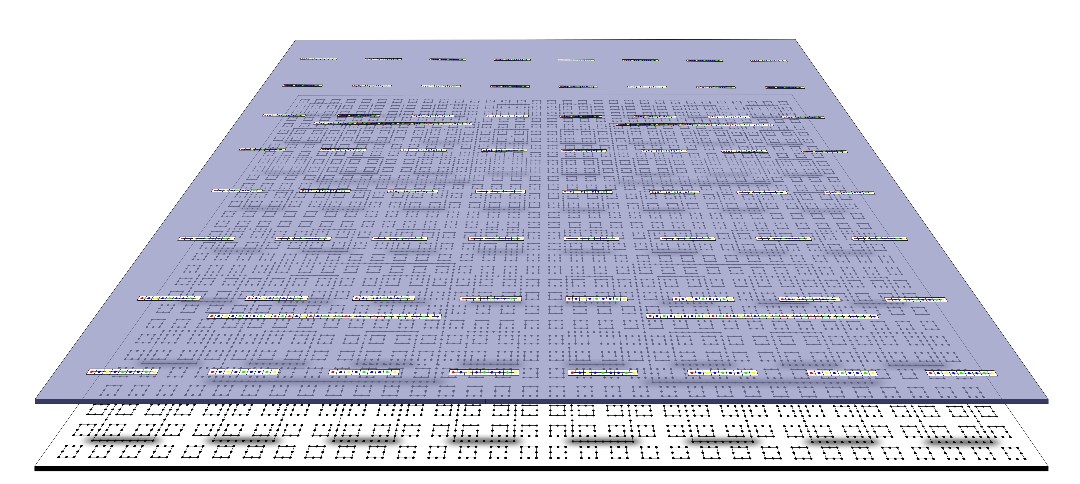# An Undecidable Quantum Physics Problem

This is cool: In today’s Nature, Toby Cubitt, David Perez-Garcia, and Michael Wolf published a paper, “Undecidability of the spectral gap.” A short writeup is in Nature News, and an extended paper is on arXiv. It shows a problem in quantum physics–the spectral gap problem–to be undecidable by reducing the halting problem to it.

In the Nature News story, the first author is quoted as saying, “I think it’s fair to say that ours is the first undecidability result for a major physics problem that people would really try to solve.” Is that true?

Note that the article also links the undecidability result to an independence result: “Our results imply that for any consistent, recursive axiomatisation of mathematics, there exist specific Hamiltonians for which the presence or absence of a spectral gap is independent of the axioms.” In order to get this consequence, you’d reduce the problem to provability/refutability in your favorite axiom system $$T$$: Give a computable function $$F$$ with the following property: If $$i$$ is an instance of the problem, $$F(i)$$ is a formula in the language of $$T$$ such that if $$T \vdash F(i)$$ then $$i$$ is a positive instance, and if $$T \vdash \lnot F(i)$$ then it is a negative instance.  If $$T$$ decided all the $$F(i)$$, searching through all theorems of $$T$$ will eventually yield either $$F(i)$$ or $$\lnot F(i)$$, and so provide a decision procedure for the problem. If the problem is undecidable, that can’t happen, so at least one $$F(i)$$ must be independent of $$T$$. (In fact, infinitely many must be, for any finite number could be treated as special cases before running the infinite search.)  But you do have to give this coding of instances of the problem. (Just for a couple of simple examples, for the halting problem and a sound arithmetical theory, $$F(i)$$ would be the standard description of “Turing machine with index $$i$$ halts on input $$i$$”; for Hilbert’s 10th problem, given a Diophantine equation $$p(\vec x) = 0$$, $$F(p)$$ would be $$\exists \vec x p(\vec x) = 0$$.  See also the paper by Björn Poonen they cite in support of their claim, esp. p. 2.)

Unless I missed something, the authors haven’t done this. So in what sense have they shown that “there exist specific Hamiltonians for which the presence or absence of a spectral gap is independent”? To use the argument above, when you have the halting problem reduced to your new decision problem, you could just take the description of “TM $$i$$ halts on input $$i$$” as your $$F(i)$$.  This will have the required property for whichever instances of your decision problem the halting problem instances reduce to.  But this isn’t quite like actually giving a method for directly coding the physical problems in arithmetic, or exhibiting a sentence of arithmetic that says “quantum many-body model $$i$$ is gapped.”

Note also that the coding $$F(i)$$ depends on the axiom system, so the order of the quantifiers matters: for each axiom system, there will be possibly different encodings of the decision problem with the required property; and it’s not the case that there are instances of the decision problem that are independent of (and hence unsolvable by) any axiom system.  You can always add $$F(i)$$ to your $$T$$ for a true instance $$i$$, or $$\lnot F(i)$$ for a false instance, and this will yield a new axiom system which decides that instance in the sense given above.  In fact you can even add $$\lnot F(i)$$ for a true instance (if $$F(i)$$ is independent and doesn’t happen to be $$\Sigma^0_1$$)!  Then you’ll get an unsound axiom system that will decide that instance incorrectly, and you’ll have to find a different coding.

I of course have no idea if the problem shown undecidable, or the features of the problem used in the reduction of the halting problem, are actually physically interesting.  It may well be that the physically interesting cases of the problem are decidable. Certainly one can decide at least some specific instances, and perhaps all instances that “occur in nature.” But IANAP.

tl;dr interesting “real-world” example of undecidability result physicists actually care about, not an interesting independence result.

## 3 thoughts on “An Undecidable Quantum Physics Problem”

1.boufelix says:

For sure it is now the first result related to physics.
http://dx.doi.org/10.1016/0001-8708(81)90001-3
http://dx.doi.org/10.1016/0003-4843(79)90021-4
And I guess it is also worth mentioning the book “Computability in analysis and physics” by M. B. Pour-El and J. I. Richards (and perhaps also https://books.google.es/books?id=wA8HMqElzWkC )

Yesterday I read some short summary in spanish of that paper (I have not looked at the paper), and there there is a huge misunderstanding of the difference between “undecidable in the sense of uncomputable problem” and “undecidable in the sense of statement independent from the chosen axioms”.

1.Richard Zach says:

You mean “not”? Not “now”? Right?

But is it the first “major” such problem?

1.boufelix says:

Sure, it is “not”.

Hard to say what is a “major” problem (I consider quite important that to know that you cannot compute the unique solutions in general, which is a weakening of the result in http://dx.doi.org/10.1016/0001-8708(81)90001-3 )